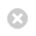Close

UCL Great Ormond Street Institute of Child Health

HomeGreat Ormond Street Institute of Child Health# Confidence Intervals for a Median

Confidence intervals were previously given with sample mean values. These intervals gave a measure of how precisely the sample estimate approximated the population value. The width of the interval depended on:

• Variability of the data
• Size of the sample

It is possible to similarly obtain a confidence interval for a sample median, the interpretation of which is directly comparable to that for the mean. However, because of the lack of distributional assumptions, it may not be possible to obtain an exact 95% confidence interval for the median.

Standard errors cannot be calculated for distribution free statistics. However, confidence intervals can be calculated and have the same interpretation; i.e., they consist of the range of population values with which the sample is compatible.

The confidence limits are not necessarily symmetric around the sample estimate (as is the case when standard errors are used to construct the confidence intervals).

The confidence limits are given by actual values in the sample. We choose which values using the following formulae:For example:

If there are 20 values in the sample:

The median is between the 10th and 11th highest ordered measurements

The 95% confidence interval for the median is given by the values ranked:

10-4.38 = 5.62 and 1+10+4.38 =15.38

Of course, there are no 5.62 and 15.38 ranked values, so we choose the nearest ranks to these and have an APPROXIMATE 95% confidence interval for the median. For the 20 values this will be the 6th to the 15th ranked values.

Example:

Consider the differences in temperature between the start and end of surgery for the 12 patients undergoing percutaneous surgery.The median difference is halfway between the 6th and 7th largest values i.e., between -0.9 and -0.7 = -0.8

There are 12 values and hence:So an approximate 95% confidence interval is given by the 3rd and 10th ranked values (-3.2, -0.2), compared to a 95% confidence interval for the mean which would be (-2.49, -0.57).